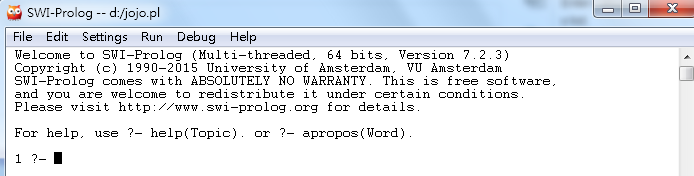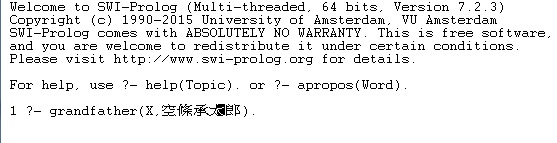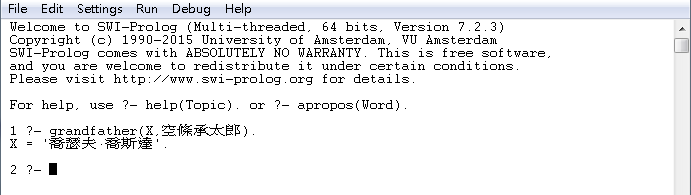# Prolog教學-基本關係判斷-誰是爺爺？Prolog厲害的地方就是可以透過我們給予的資訊，自己去找關連性，

(這裡請任意挑自己喜歡作品的名稱放)

``````father('喬瑟夫·喬斯達','荷莉').
mother('絲吉Q','荷莉').
mother('荷莉','空條承太郎').

grandfather(X,Y):-
father(X,Z),father(Z,Y);
father(X,Z),mother(Z,Y).

grandmother(X,Y):-
mother(X,Z),father(Z,Y);
mother(X,Z),mother(Z,Y).

``````

father('喬瑟夫·喬斯達','荷莉').  意思是 '喬瑟夫·喬斯達' 是 '荷莉' 的爸爸

``````grandfather(X,Y):-
father(X,Z),father(Z,Y);
father(X,Z),mother(Z,Y).``````

``grandfather(X,Y):-``

``father(X,Z),father(Z,Y);``

``father(X,Z),mother(Z,Y).``?- 後面黑色框框就是可以輸入問題的地方，我們可以把以下問題先貼進去

``````grandfather(X,空條承太郎).
``````

如下圖，再按下Enter把問題送出。就會發現Prolog回答我們X是多少，也就是'空條承太郎'的爺爺是誰發現Prolog會告訴我們答案是喬瑟夫·喬斯達。

Prolog就非常有用了！